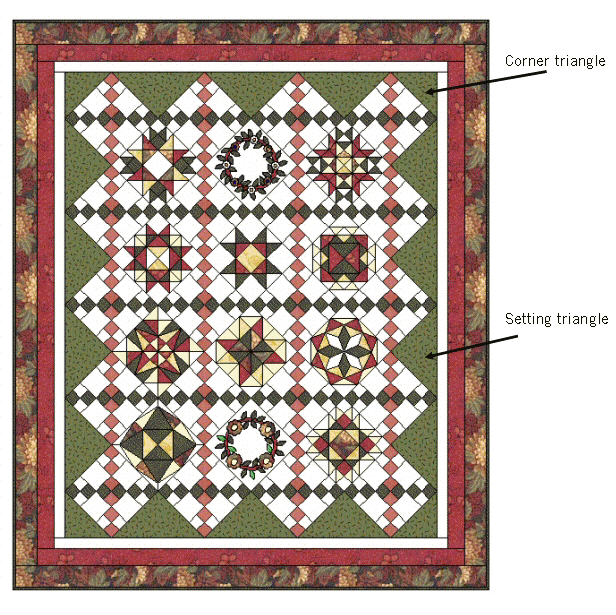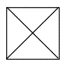In a diagonally set quilt, blocks are set on point with the sides of the blocks running diagonally at a 45° angle to the sides of the quilt as shown in the illustration below.  Large setting triangles along the sides of the quilt and smaller triangles at the four corners fill in around the blocks and complete the quilt.  For this setting, blocks are sewn together in diagonal rows (adding setting or corner triangles at the ends of each row as needed).Calculating these triangles can be a little tricky if you're not familiar with the technique.  Please note that setting and corner triangles need to be cut differently because of grain lines.For setting triangles:  Use a large square and cut twice on the diagonal--the long side of each of these triangles is on the straight of grain.  You will cut 4 triangles from each square and need to do some simple math to determine how many setting triangles you need for your particular quilt.  To determine the size of the square, use this simple formula: Finished Block Size x 1.41 = Finished Diagonal + 1.25 =Size of Square to Cut (round off to the nearest 1/8")For corner triangles:  Use a large square and cut once on the diagonal--the short sides of each of these triangles is on the straight of grain.  You will cut two triangles from each square--for the 4 corners of your quilt you will need to cut two squares the same size and cut into 4 triangles.  To determine the size of the square, use this simple formula: Finished Block Size x 1.41 = Finished Diagonal divided by 2 + .875" = Size of Square to Cut (round off to the nearest 1/8")

 Cutting Chart for Common Setting and Corner Triangles Finished Block Size Finished Diagonal Size to Cut Square for: Setting TrianglesCorner Triangles6" 8.5" 9.75" 5.125" 8" 11.375" 12.625" 6.625" 9" 12.75" 14.0" 7.25" 10" 14.125" 15.375" 8.0" 12" 17.0" 18.25" 9.375" 14" 19.75" 21.0" 10.75" 15" 21.25" 22.5" 11.5"

 Decimal Conversion Chart 1/8 1/4 3/8 1/2 5/8 3/4 7/8 .125 .25 .375 .5 .625 .75 .875